## Annotating Dates for the xaxis in SGPLOT

Hi, I am no longer able to use the old "angle" command that I used to use in an axis statement in gplot. sgplot requires annotation to be able to angle our tick marks on the xaxis. I found some code on the SAS site but it doesn't seem to work with dates. Has anyone ever applied annotation in sgplot to angle date format tick marks upward about 45 degrees read from left to right? If so, can you share your code, please. I have tried a variety of options in proc template and a few in sgplot to no avail.

1 ACCEPTED SOLUTION

Accepted Solutions

## Re: Annotating Dates for the xaxis in SGPLOT

Had another go using annotate, have a look before. Had to use RANGES to get the desired thickmarks. Give it a try.

``````data ts;
do year = 2005 to 2015;
do month = 1 to 12 by 2;
myDate = mdy(month, 1, year);
value = ceil(ranuni(123) * 5);
y0=0;

if month = 1 then do;
myDate_c = put(myDate, monyy.);
end;
else do;
call missing(myDate_c);
end;

if myDate > "01aug2015"d then do;
leave;
end;

row + 1;
output;
end;
end;

format
myDate date9.
value nlnum12.
;
run;

%SGANNO

data ts_anno;
set ts(keep=myDate myDate_c );
where myDate_c not is missing;

%SGTEXT(
LABEL=myDate_c
, ANCHOR= "bottom"
, TEXTCOLOR="red"
, X1SPACE="datavalue"
, X1=myDate
, y1space="WALLPERCENT"
, y1=-8
, LAYER="front"
, rotate=45
)
run;

series x=myDate y=value;
xaxis type=time
ranges=(
"01JAN2005"d -  "01jul2015"d
)
display=(novalues nolabel)
grid
;
yaxis
min=0 max=5
grid
;
run;
``````
14 REPLIES 14

## Re: Annotating Dates for the xaxis in SGPLOT

Have a look through this blog:

http://blogs.sas.com/content/graphicallyspeaking/

It has examples of every possible graphing technique you can imagine.  Try searching for annotate.

## Re: Annotating Dates for the xaxis in SGPLOT

This is a good article. Unfortunately, it still does not address my question. I am able to format the tick mark date values in the same ways suggested by the author. Unfortunately, I work at a place that wishes me to format them angled diagonally read from right to left like so: /   /   /   /.. None of the functions presented here can accomplish this nor can they be adapted to do this as far as I know. Any other suggestions would be greatly appreciated.

## Re: Annotating Dates for the xaxis in SGPLOT

CAn you perhaps provide an example picture of what you would want it to look like, I don't get it from a string of slashes.

## Re: Annotating Dates for the xaxis in SGPLOT

Sure, I'll attach the graph. These things have been done in excel for years, and while I can do the same thing with SAS, it doesn't turn out exactly like this graph on the xaxis. With GPLOT it used to be easy because I would add a statement such as: axis1 value= (h=1 angle= 45) label=(angle=0 " ") order=("01JAN05"d to "01AUG15"d by year); and it would automatically give me what I needed. Unfortunately, GPLOT leaves a lot of white space if the series finishes at any month other the January. SGPLOT and Proc Template are able to solve this problem, but unfortunately neither of them accept the angle command. There's a variety of rotate options for the tick values but they only go vertical or diagonal going down read from right to left. Check out the attachment.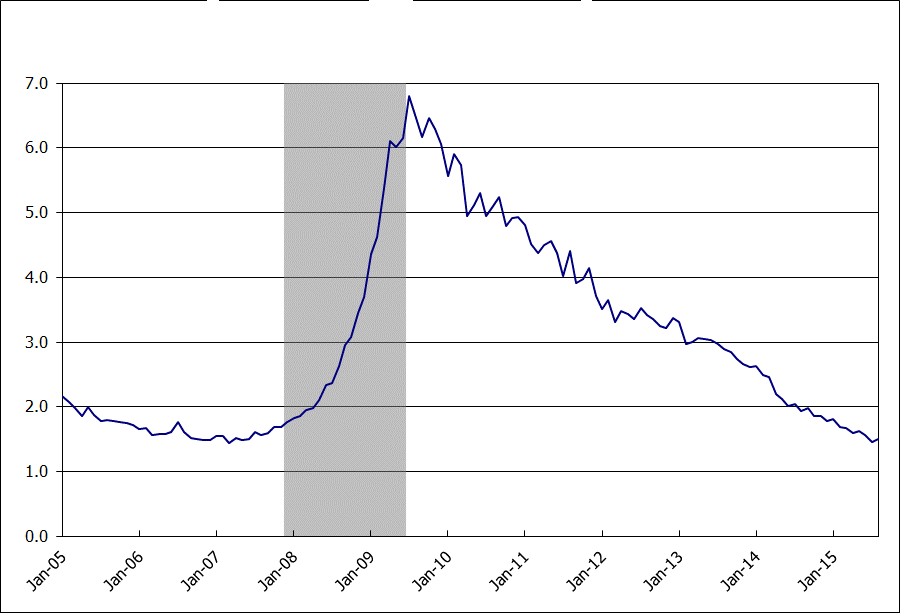## Re: Annotating Dates for the xaxis in SGPLOT

Yes, I see.  As far as I can tell there isn't a way to have the ticks go left to right up at 45 degree's, only down 45 degrees:

#### TICKVALUEROTATION=DIAGONAL | VERTICAL

specifies how the tick values are rotated on the X and X2 axes.

 DIAGONAL rotates the tick values to a 45-degree diagonal position. The X labels read left to right in a downward direction. The X2 labels read left to right in an upward direction. VERTICAL rotates the labels to a 90-degree vertical position. The labels are always drawn from bottom to top.
 Default DIAGONAL Restriction This option is valid for XAXISOPTS= and X2AXISOPTS= only. Interaction The TICKVALUEFITPOLICY= option must be set to ROTATE or ROTATEALWAYS for this option to have any effect.

There's an idea for it:

https://communities.sas.com/t5/SASware-Ballot-Ideas/ODS-GTL-Allow-tick-value-rotation-on-x-axis-to-b...

Unless anyone else has any information?

## Re: Annotating Dates for the xaxis in SGPLOT

I assume you are somewhere in Europe, Since we label tick marks like this / /, but SGPLOT would do it like this \ \.

Have a  look at the sample code below. There is a simple version using the TEXT plot statement to label and another version using GTL where we have more control.

``````data ts;
do year = 2015;
do month = 1 to 12;
myDate = mdy(month, 1, year);
value = ceil(ranuni(123) * 5);
y0=-0.5;
myDate_c = put(myDate, ddmmyyp10.);
output;
end;
end;
format
myDate date9.
value nlnum12.
;
run;

proc sgplot data=ts;
series x=myDate y=value;
text x=mydate y=y0 text=myDate_c /
rotate=45
;
xaxis display=(NOLINE NOVALUES) ;
refline 0;
run;
ods path
(prepend) work.mytemplates (update)
;

proc template;
define statgraph sgdesign;
dynamic _MYDATE _VALUE  _Y0A;
begingraph;
entrytitle halign=center 'Some title';
entryfootnote halign=left 'some footnote';
layout lattice /
rows=2
rowgutter=0
rowweights=(0.88 0.12)
;
layout overlay /
xaxisopts=( display=(ticks))
yaxisopts=(
display=(ticks tickvalues label)
linearopts=( viewmin=0 viewmax=5 )
)

;
seriesplot x=_MYDATE y=_VALUE /
name='series' connectorder=xaxis
;
endlayout;
layout overlay / walldisplay=none
yaxisopts=( display=none)
xaxisopts=( display=none)
;
textplot x=_MYDATE y=_Y0A text=myDate_c / rotate=45;
endlayout;
endlayout;
endgraph;
end;
run;

proc sgrender data=WORK.TS template=sgdesign;
dynamic _MYDATE="MYDATE" _VALUE="VALUE"  _Y0A="Y0";
run;``````

Bruno

## Re: Annotating Dates for the xaxis in SGPLOT

Thanks, Bruno. This seems to be closest to what I need. What is your suggestion if I want to display my entire series say from January 2005 until August 2015, but I only want to display January Tick Values of each year? Right now I'm trying to do it without the date facing the proper way using:

xaxisopts=(label=" " offsetmax=0 type=time timeopts=(tickvaluelist=("01JAN05"d "01JAN06"d "01JAN07"d "01JAN08"d

"01JAN09"d "01JAN10"d "01JAN11"d "01JAN12"d "01JAN13"d "01JAN14"d "01JAN15"d) viewmin="01JAN05"d max="01AUG15"d));

yaxisopts=(label=" " type=linear linearopts=(tickvaluelist=(0 1000 2000 3000 4000 5000 6000) viewmin=0 viewmax=6000));

seriesplot x='date'n y='jo_sa'n / primary=true lineattrs=( color=blue pattern=solid thickness=1.5);

Is there somthing I can do in GTL that is the equivalent of the following in sgplot, for some reason I didn't have to specify an interval when I used "ranges", it just did January automatically and included the entire series up to August 2015:

xaxis label=' ' ranges=("01JAN05"d - "01AUG15"d) tickvalueformat=monyy. ;

Basically, how can I adapt your gtl code to include just the tick marks and diagonally upward formatted labels for January of each year from 2005 until now. I don't want it to cut off the series in January of this year, but I also wouldn't want to include January 16 as a tick mark (this leaves a lot of white space on the graph).

## Re: Annotating Dates for the xaxis in SGPLOT

Jibra

find below a changed example. The labeling of the X-axis is done with the TEXT / TEXTPLOT statement, so you can control the value of the variable that is used with the TEXT / TEXTPLOT to only label the points you want. The tickmarks from the SERIESPLOT are done automatically, and for this example they seem to fit, with what you need.

According to the doc RANGES is used for broken axis

``````data ts;
do year = 2005 to 2015;
do month = 1, 4, 7, 10;
myDate = mdy(month, 1, year);
value = ceil(ranuni(123) * 5);
y0=-0.5;
if month = 1 then do;
myDate_c = put(myDate, monyy.);
end;
else do;
call missing(myDate_c);
end;
if myDate > "01jan2015"d then do;
leave;
end;
output;
end;
end;
format
myDate date9.
value nlnum12.
;
run;

proc sgplot data=ts;
series x=myDate y=value;
text x=mydate y=y0 text=myDate_c /
rotate=45
;
xaxis display=(NOLINE NOVALUES) ;
refline 0;
run;

ods path
(prepend) work.mytemplates (update)
;

proc template;
define statgraph sgdesign;
dynamic _MYDATE _VALUE  _Y0A;
begingraph;
entrytitle halign=center 'Some title';
entryfootnote halign=left 'some footnote';
layout lattice /
rows=2
rowgutter=0
rowweights=(0.88 0.12)
;
layout overlay /
xaxisopts=( griddisplay=on display=(ticks))
yaxisopts=(
display=(ticks tickvalues label )
linearopts=( viewmin=0 viewmax=5 )
)

;
seriesplot x=_MYDATE y=_VALUE /
name='series' connectorder=xaxis
;
endlayout;
layout overlay / walldisplay=none
yaxisopts=( display=none)
xaxisopts=( display=none)
;
textplot x=_MYDATE y=_Y0A text=myDate_c / rotate=45;
endlayout;
endlayout;
endgraph;
end;
run;

proc sgrender data=WORK.TS template=sgdesign;
dynamic _MYDATE="MYDATE" _VALUE="VALUE"  _Y0A="Y0";
run;``````

Bruno

## Re: Annotating Dates for the xaxis in SGPLOT

Thank you Bruno. Your code works perfectly to make the tick mark values diagonal in gtl. Unfortunately, I am back where I was with simple gplot. I want the series to graph line to begin immediately on the y axis and end at the edge fo the chart. I had been able to angle my tickmarks with gplot before but when the time series input extended past January 15 to August data, I was left with a chunk of white space and an undesired January 16 tick marks. There was always a bit of white after the end of the series. That's why I started to look at other options and encountered all the angle issues with sgplot and proc template. In adapting your code, i encountered white space on both sides (see attached). I tried to counter that with the offset to 0 and linearopts to 0 functions, and I ended up with the same white space on the right side of the graph and losing a portion of my first tick value label. Is there an easy way to adjust your code to mimic the graph I attached yesterday? This code has been a great education.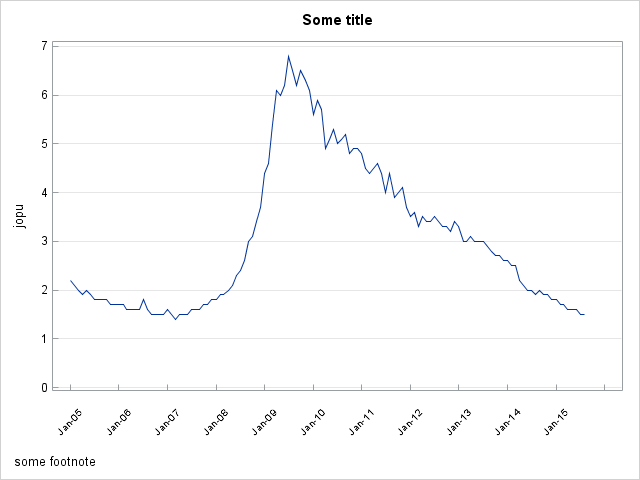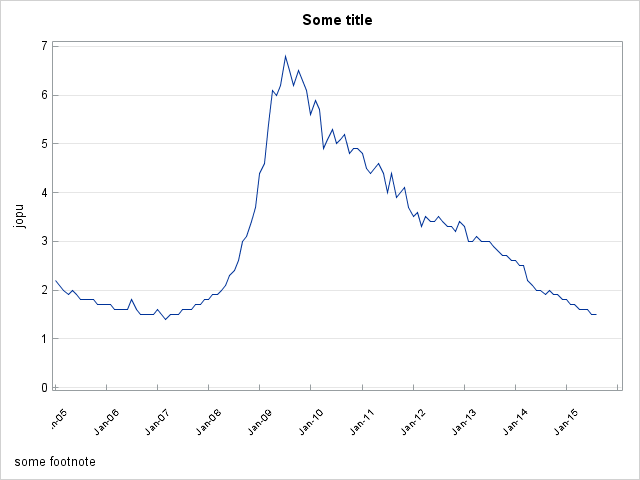## Re: Annotating Dates for the xaxis in SGPLOT

At the moment I can not help much more. ODS Graphics also supports annotate, I can imagine, that we can do something like this with annotate, and maybe go away from the axis type time. to get exactly what you need.

Bruno

## Re: Annotating Dates for the xaxis in SGPLOT

Had another go using annotate, have a look before. Had to use RANGES to get the desired thickmarks. Give it a try.

``````data ts;
do year = 2005 to 2015;
do month = 1 to 12 by 2;
myDate = mdy(month, 1, year);
value = ceil(ranuni(123) * 5);
y0=0;

if month = 1 then do;
myDate_c = put(myDate, monyy.);
end;
else do;
call missing(myDate_c);
end;

if myDate > "01aug2015"d then do;
leave;
end;

row + 1;
output;
end;
end;

format
myDate date9.
value nlnum12.
;
run;

%SGANNO

data ts_anno;
set ts(keep=myDate myDate_c );
where myDate_c not is missing;

%SGTEXT(
LABEL=myDate_c
, ANCHOR= "bottom"
, TEXTCOLOR="red"
, X1SPACE="datavalue"
, X1=myDate
, y1space="WALLPERCENT"
, y1=-8
, LAYER="front"
, rotate=45
)
run;

series x=myDate y=value;
xaxis type=time
ranges=(
"01JAN2005"d -  "01jul2015"d
)
display=(novalues nolabel)
grid
;
yaxis
min=0 max=5
grid
;
run;
``````

## Re: Annotating Dates for the xaxis in SGPLOT

Bruno-your last code is perfect for what I want; when I run it on its own it gives me exactly what I want. After I adapted it with my own using my own table as data source and my own date format, I lost all the tick marks except for two (see image). Where did I go wrong? Nevertheless, this is he absolute best code for angling tickmarks in any of the plotting sas functions. Thank you!!!!

```proc sql noprint;
create table CP_JO as
SELECT
round((a.value/b.jo_sa), 0.1) as jopu,
a.year,
a.month,
MDY(b.month, 01, b.year) as sasdate,
6000 as x1,
MDY(b.month, 01, b.year) format=daslt.  as date

FROM
cps a, jlt2 b
where a.month=b.month and a.year=b.year
order by year, month;
quit;

data bruno;
set cp_jo;
do year = 2005 to 2015;
do month = 1 to 12 by 2;
sasdate = mdy(month, 1, year);
y0=0;

if month = 1 then do;
myDate_c = put(sasdate, daslt.);
end;
else do;
call missing(myDate_c);
end;

if myDate > "01aug2015"d then do;
leave;
end;

row + 1;
output;
end;
end;

format
sasdate date9.
jopu nlnum12.
;
keep year month sasdate jopu y0 x1 mydate_c row;
run;

%SGANNO

data jp_anno;
set bruno(keep=sasdate myDate_c );
where myDate_c not is missing;

%SGTEXT(
LABEL=myDate_c
, ANCHOR= "bottom"
, TEXTCOLOR="black"
, X1SPACE="datavalue"
, X1=sasdate
, y1space="WALLPERCENT"
, y1=-8
, LAYER="front"
, rotate=45
)
run;

proc sgplot data=cp_jo pad=(bottom=10pct) sganno=jp_anno noautolegend;
band y=x1 lower="01DEC07"d upper="01JUN09"d / transparency=.8 fillattrs=(color=gray);

series x=sasdate y=jopu;
xaxis type=time
ranges=(
"01JAN2005"d - "01aug2015"d
)
display=(novalues nolabel )

;

yaxis
grid
;
YAXIS LABEL = ' ' GRID VALUES = (0 TO 7 BY 1);
run;
```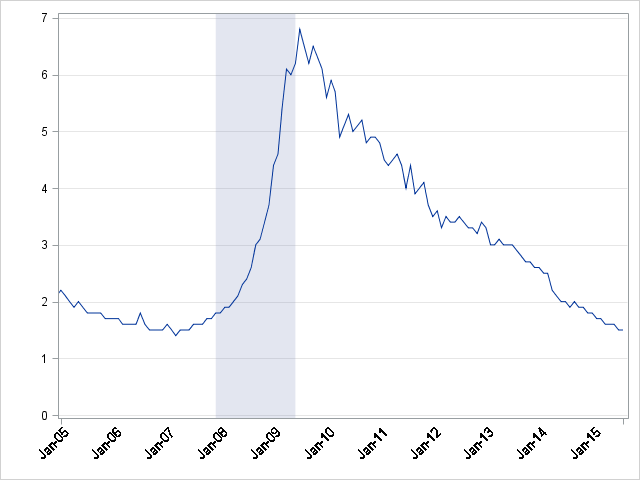## Re: Annotating Dates for the xaxis in SGPLOT

What is the time Interval of your data? Is it monthly data? Are some months missing?

## Re: Annotating Dates for the xaxis in SGPLOT

Basically, I have data that is in a monthly interval. However, I need my graph to display the rate associated with the y axis in yearly intervals. Proc GPLOT and Proc Template were either cutting off the series line at either Jan15 or adding a bunch of white space and another tick mark for January 16. Your code, which I designated as the solution was adaptable to get as close as possible to what I need. In my first proc sql statement, I already had my months in numeric formats and I kept them all, but I did my other date formats here, and added the columns I need for annotations. In your data step, I pulled everything I need from the table I created in sql, did my conversion of the January sasdates to my own format, made sure all other months were missing--not sure why we are adding a row number, but I kept that. Next I did the annotation exactly as you coded. Then the Plot gave me almost exactly what I wanted. I hate to be greedy, but what are the functions in sgplot that would let us move the tickmarks into the graph? GTL has tickstyle=inside, but I couldn't find an equivalent for sgplot. Also, is there a way to change the colors of the grid lines? Thank you very much for all your assistance.

My final code:

proc sql noprint;

create table CP_JO as

SELECT

round((a.value/b.jo_sa), 0.1) as jopu,

b.year

b.month,

MDY(b.month, 01, b.year) format=date9. as sasdate,

6000 as r1,

0 as y,

MDY(b.month, 01, b.year) format=daslt. as date

FROM

cps a, jlt2 b

where a.month=b.month and a.year=b.year

order by year, month;

quit;

data bruno;

set cp_jo;

if year>2004;

if month = 1 then do;

myDate_c = put(sasdate, daslt.);

end;

else do;

call missing(myDate_c);

end;

row + 1;

output;

;

keep year month sasdate jopu y0 r1 mydate_c row;

run;

%SGANNO

data jp_anno;

set bruno(keep=sasdate myDate_c );

where myDate_c not is missing;

%SGTEXT(

LABEL=myDate_c

, ANCHOR= "bottom"

, TEXTCOLOR="black"

, X1SPACE="datavalue"

, X1=sasdate

, y1space="WALLPERCENT"

, y1=-8

, LAYER="front"

, rotate=45

)

run;

proc sgplot data=cp_jo pad=(bottom=10pct) sganno=jp_anno noautolegend;

band y=r1 lower="01DEC07"d upper="01JUN09"d / transparency=.8 fillattrs=(color=gray);

series x=sasdate y=jopu;

xaxis type=time

ranges=(

"01JAN2005"d - "01aug2015"d

)

display=(novalues nolabel )

;

yaxis

grid

;

YAXIS LABEL = ' ' GRID VALUES = (0 TO 7 BY 1);

run;

Here's an abbreviated section of the bruno table from the data step above:

 jopu year month sasdate r1 myDate_c row 2.2 2005 1 1-Jan-2005 6000 Jan-05 1 2.1 2005 2 1-Feb-2005 6000 2 2 2005 3 1-Mar-2005 6000 3 1.9 2005 4 1-Apr-2005 6000 4 2 2005 5 1-May-2005 6000 5 1.9 2005 6 1-Jun-2005 6000 6 1.8 2005 7 1-Jul-2005 6000 7 1.8 2005 8 1-Aug-2005 6000 8 1.8 2005 9 1-Sep-2005 6000 9 1.8 2005 10 1-Oct-2005 6000 10 1.7 2005 11 1-Nov-2005 6000 11 1.7 2005 12 1-Dec-2005 6000 12 1.7 2006 1 1-Jan-2006 6000 Jan-06 13 1.7 2006 2 1-Feb-2006 6000 14 1.6 2006 3 1-Mar-2006 6000 15 1.6 2006 4 1-Apr-2006 6000 16 1.6 2006 5 1-May-2006 6000 17 1.6 2006 6 1-Jun-2006 6000 18

And here is what the anno table looks like:

 ANCHOR FUNCTION LABEL LAYER TEXTCOLOR X1SPACE Y1SPACE ROTATE X1 Y1 bottom TEXT Jan-05 front black datavalue WALLPERCENT 45 16437 -8 bottom TEXT Jan-06 front black datavalue WALLPERCENT 45 16802 -8 bottom TEXT Jan-07 front black datavalue WALLPERCENT 45 17167 -8 bottom TEXT Jan-08 front black datavalue WALLPERCENT 45 17532 -8 bottom TEXT Jan-09 front black datavalue WALLPERCENT 45 17898 -8 bottom TEXT Jan-10 front black datavalue WALLPERCENT 45 18263 -8 bottom TEXT Jan-11 front black datavalue WALLPERCENT 45 18628 -8 bottom TEXT Jan-12 front black datavalue WALLPERCENT 45 18993 -8 bottom TEXT Jan-13 front black datavalue WALLPERCENT 45 19359 -8 bottom TEXT Jan-14 front black datavalue WALLPERCENT 45 19724 -8 bottom TEXT Jan-15 front black datavalue WALLPERCENT 45 20089 -8

And the plot is attached. I still would love to be able to move the tickmarks inside and to make the gridlines black. Much thanks. I think this thread will be a great reference.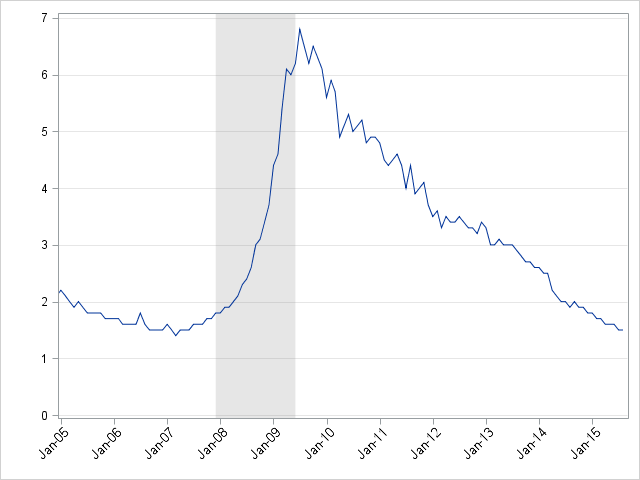Discussion stats
• 14 replies
• 5240 views
• 3 likes
• 3 in conversation